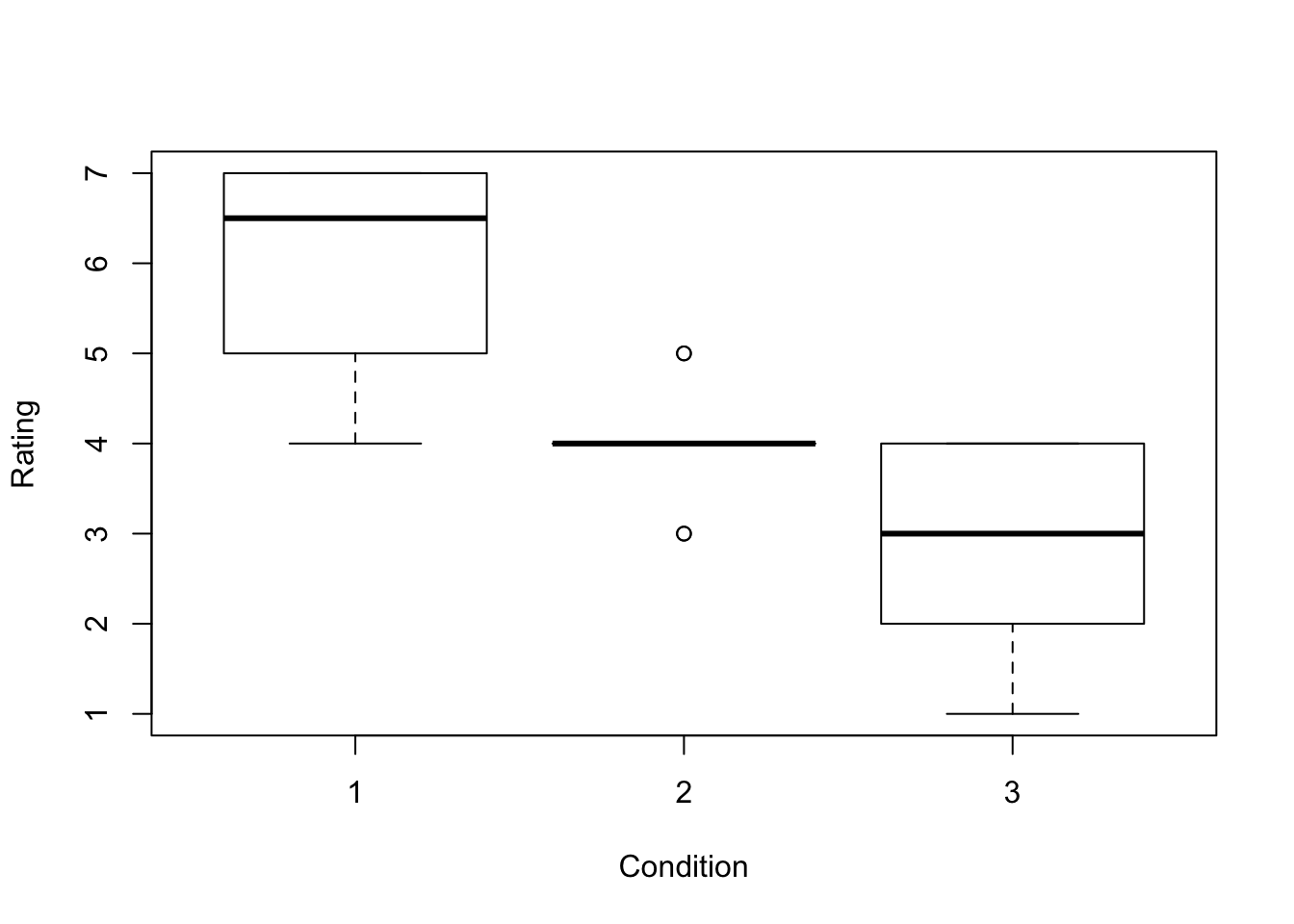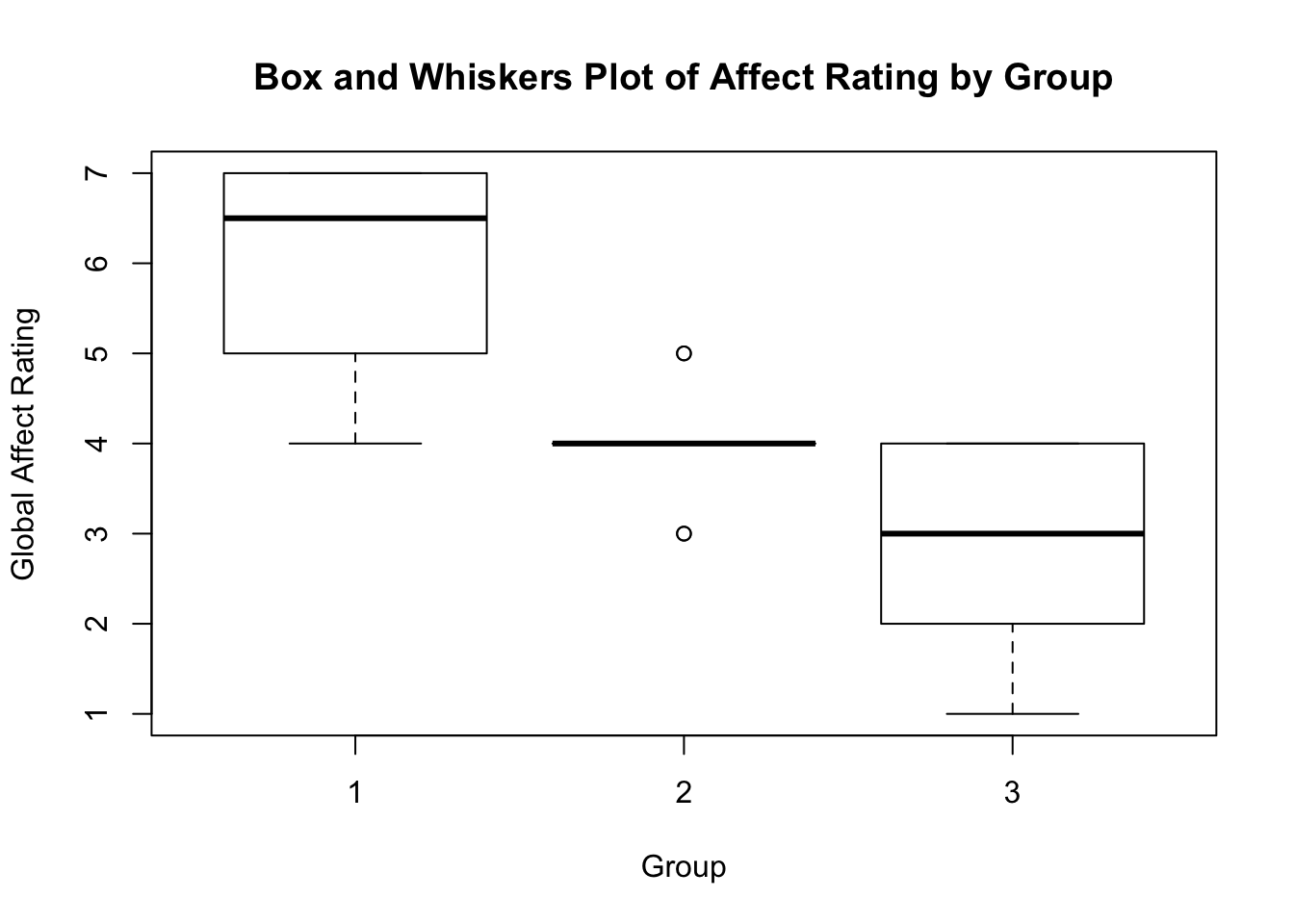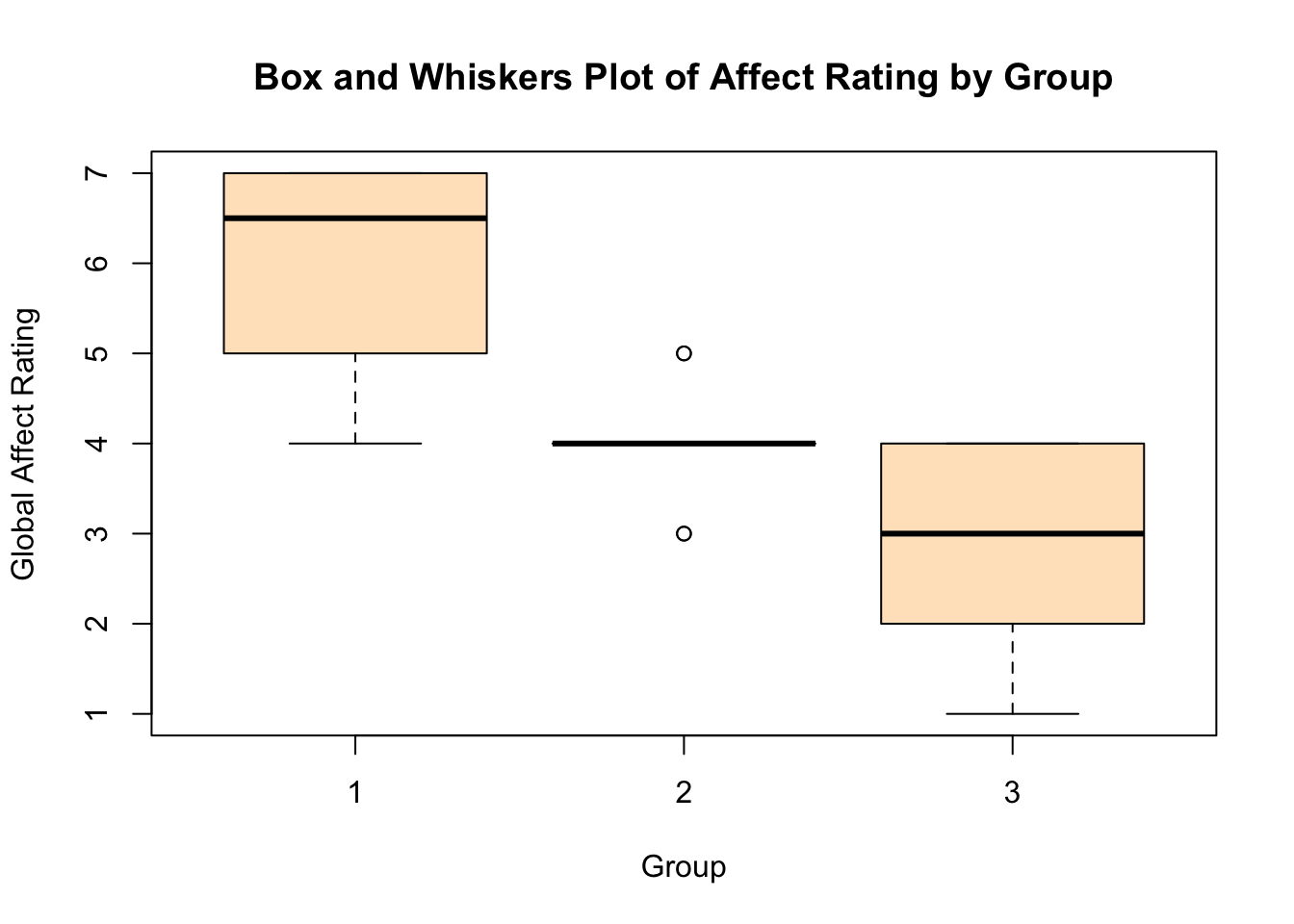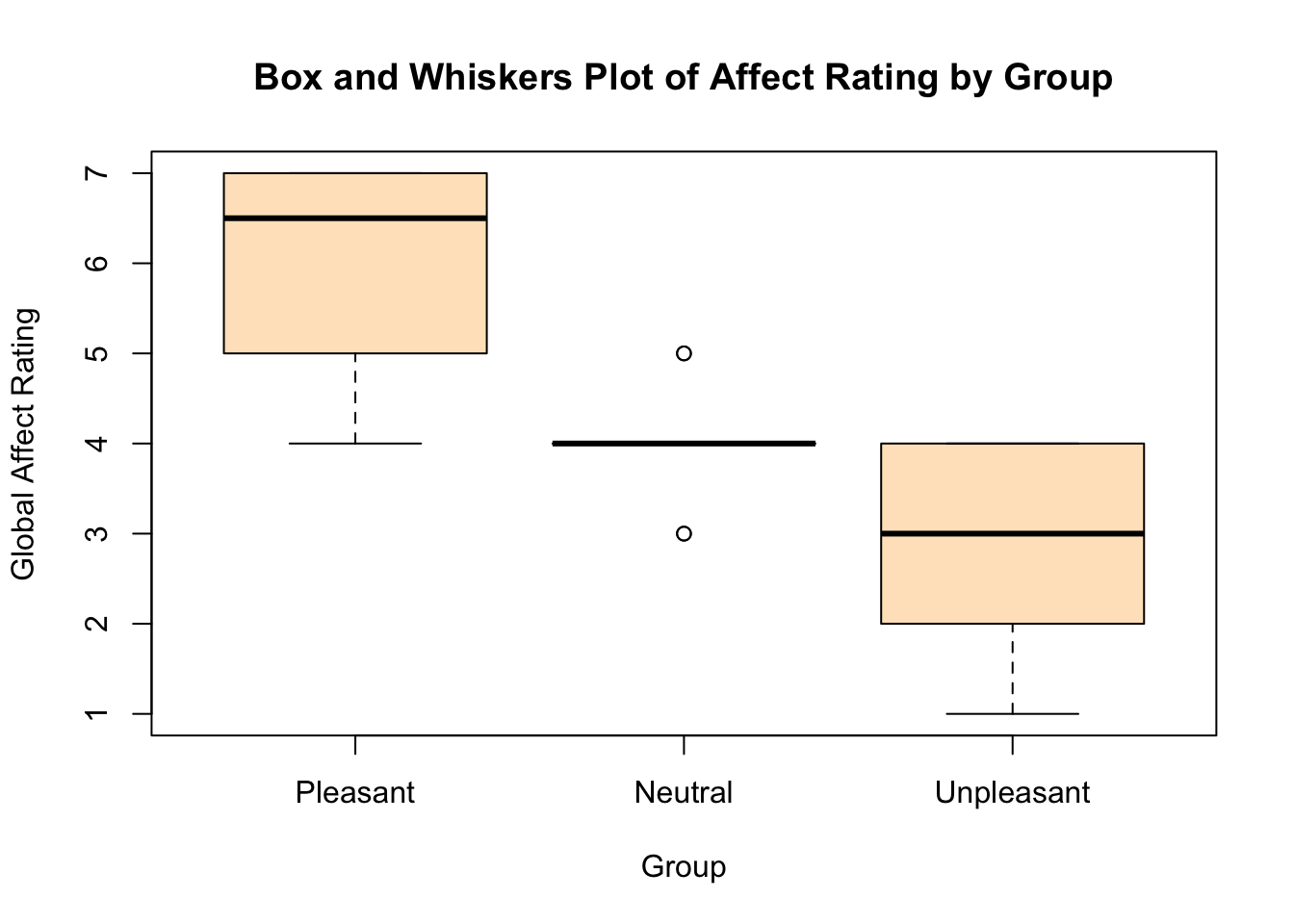# Designing Experiments and Analyzing Data: A Model Comparison Perspective (3rd edition) by Maxwell, Delaney, & Kelley

## Information about the book is available at https://designingexperiments.com

### R Code and Instructions to Accompany Chapter 3

For the Chapter 1 R code we went into considerable detail on using R, such as how to install packages, load packages, and use various functions. Note that the AMCP first needs to be installed (see the Chapter 1 page). Further note that the data sets are organized using the chapter number and table number, each separated with an underscore (e.g., chapter_3_table_1; chapter_11_table_20). For a list of data sets use `data()` with `package="AMCP"` specified as `data(package="AMCP")`.

We begin with Table 3, which is the data upon which Figure 3.1 is based.

``````library(AMCP)
data(chapter_3_table_3)
chapter_3_table_3``````
``````##    Condition Rating
## 1          1      6
## 2          1      5
## 3          1      4
## 4          1      7
## 5          1      7
## 6          1      5
## 7          1      5
## 8          1      7
## 9          1      7
## 10         1      7
## 11         2      5
## 12         2      4
## 13         2      4
## 14         2      3
## 15         2      4
## 16         2      3
## 17         2      4
## 18         2      4
## 19         2      4
## 20         2      5
## 21         3      3
## 22         3      3
## 23         3      4
## 24         3      4
## 25         3      4
## 26         3      3
## 27         3      1
## 28         3      2
## 29         3      2
## 30         3      4``````

To return the data (i.e., see what it looks like) you can simply call upon it.

``chapter_3_table_3``
``````##    Condition Rating
## 1          1      6
## 2          1      5
## 3          1      4
## 4          1      7
## 5          1      7
## 6          1      5
## 7          1      5
## 8          1      7
## 9          1      7
## 10         1      7
## 11         2      5
## 12         2      4
## 13         2      4
## 14         2      3
## 15         2      4
## 16         2      3
## 17         2      4
## 18         2      4
## 19         2      4
## 20         2      5
## 21         3      3
## 22         3      3
## 23         3      4
## 24         3      4
## 25         3      4
## 26         3      3
## 27         3      1
## 28         3      2
## 29         3      2
## 30         3      4``````

To learn the structure of the data, the `str()` function can be used. Importantly, here, we see that Condition is treated as an integer (a number).

``str(chapter_3_table_3)``
``````## 'data.frame':    30 obs. of  2 variables:
##  \$ Condition: int  1 1 1 1 1 1 1 1 1 1 ...
##  \$ Rating   : int  6 5 4 7 7 5 5 7 7 7 ...``````

The above can also be seen by using the `class()` function, which shows that Conditionition as a numeric variable.

``class(chapter_3_table_3\$Condition)``
``##  "integer"``

We can convert Condition into a factor, so that it is explicitly recognized as a grouping variable, using the `as.factor()` function and redefining the Condition variable.

``````chapter_3_table_3\$Condition <- as.factor(chapter_3_table_3\$Condition)
class(chapter_3_table_3\$Condition)``````
``##  "factor"``

A simple (box and whiskers) plot can be performed using the `plot()` function (now that Conditionition is treated as a factor).

``plot(chapter_3_table_3)``More descriptive labels and/or a title can be added

``````plot(chapter_3_table_3, ylab="Global Affect Rating", xlab="Group",
main="Box and Whiskers Plot of Affect Rating by Group")``````Equivalently, the `boxplot()` function can be used with the model specified. The “model” is simply DV~IV (here with color added to the box)

``````boxplot(Rating~Condition, data=chapter_3_table_3, ylab="Global Affect Rating", xlab="Group",
main="Box and Whiskers Plot of Affect Rating by Group", col="bisque")``````Now with names for the grouping variable

``````boxplot(Rating~Condition, data=chapter_3_table_3, ylab="Global Affect Rating", xlab="Group",
main="Box and Whiskers Plot of Affect Rating by Group", col="bisque",
names=c("Pleasant", "Neutral", "Unpleasant"))``````Now with points.

``````boxplot(Rating~Condition, data=chapter_3_table_3, ylab="Global Affect Rating", xlab="Group",
main="Box and Whiskers Plot of Affect Rating by Group", col="bisque",
names=c("Pleasant", "Neutral", "Unpleasant"))
points(Rating~Condition, data=chapter_3_table_3)``````